Help Center
1. Help Center
2. WinjiGo
3. Courses Management

# View Course Insights

The Insights page displays an overview about the course statistics, information about the learning outcomes, as well as some insights about the course members.

To view the course insights

• From the course page, click the Insights The system displays the course statistics page as in the following figure.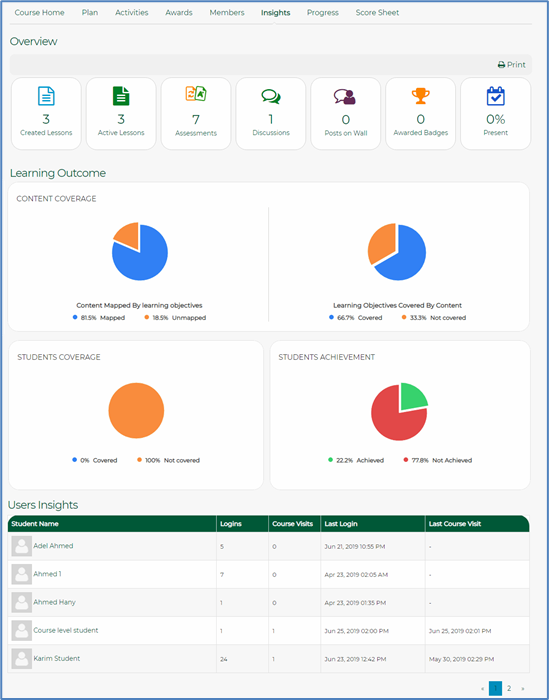The Insights page is composed of the following sections:

• Overview
• Learning Outcome
• Users Insights

### Overview: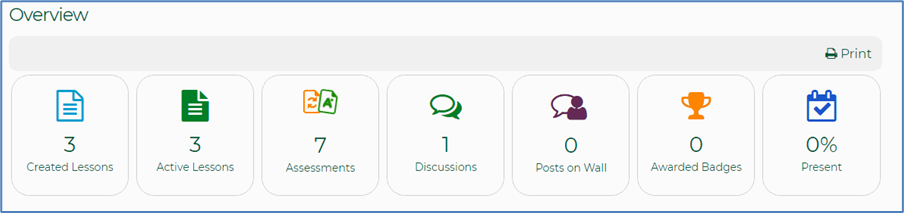The Overview section displays information about the number of created lessons, active lessons, assessments, discussions, posts on walls, awarded badges, and attendance percentage.

### Learning Outcome

The Learning Outcome section comprises the following sections:

• Content Coverage – Content Mapped by Learning Objectives
• Content Coverage – Learning Objectives Covered by Content
• Students Coverage
• Students Achievements

#### Content Coverage- Content Mapped by Learning Objectives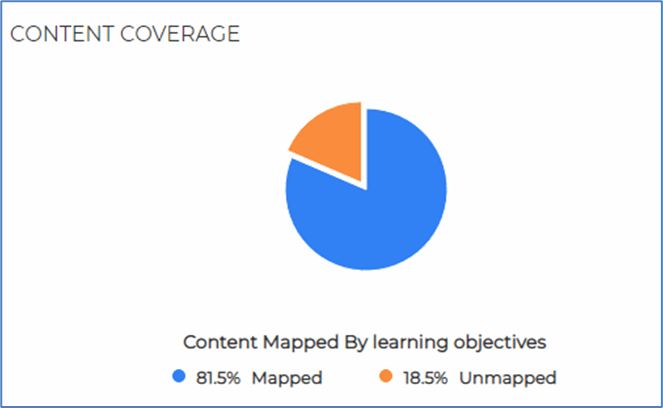This graph represents the percentage of content, added in the different courses, that was mapped to learning objectives created on the same subject and grade as the courses where the content was added. The Mapped part represents the percentage of content that was mapped to a learning objective, while the Unmapped section represents the percentage of content that was added in the courses but was not mapped to any learning objective.

Clicking the graph displays more details about each content, and the learning objective to which it was mapped, as in the following figure.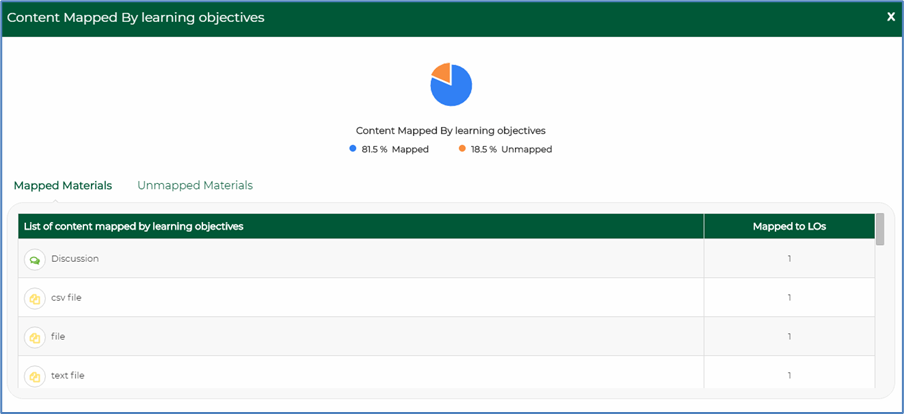#### Content Coverage - Learning Objectives Covered by Content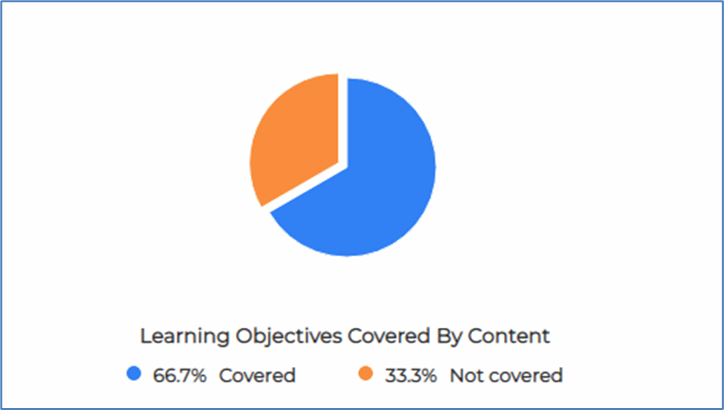This graph represents the percentage of learning objectives used across the courses, and mapped to the content added in these courses. The Covered percentage represents the percentage of Learning Objectives that were mapped to content across the courses, while the Not Covered percentage represents the percentage of Learning Objectives that exist in the organization but was not mapped to any of the content existing in the courses.

Clicking the graph displays more information about the learning objectives and the content to which they were mapped as in the following figure.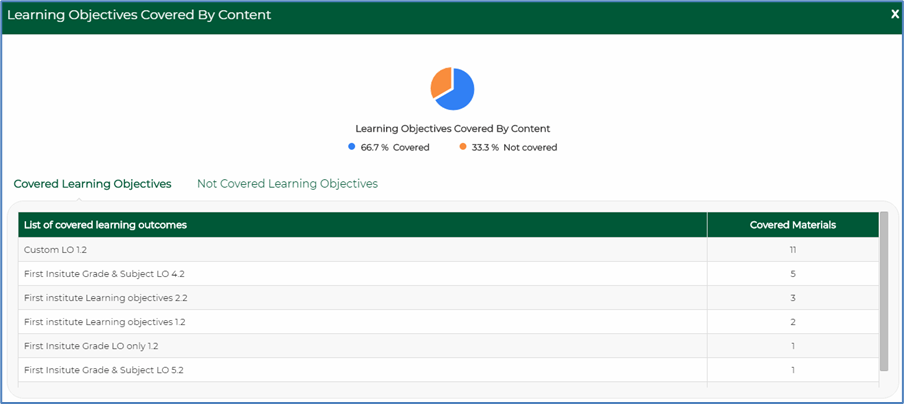#### Students Coverage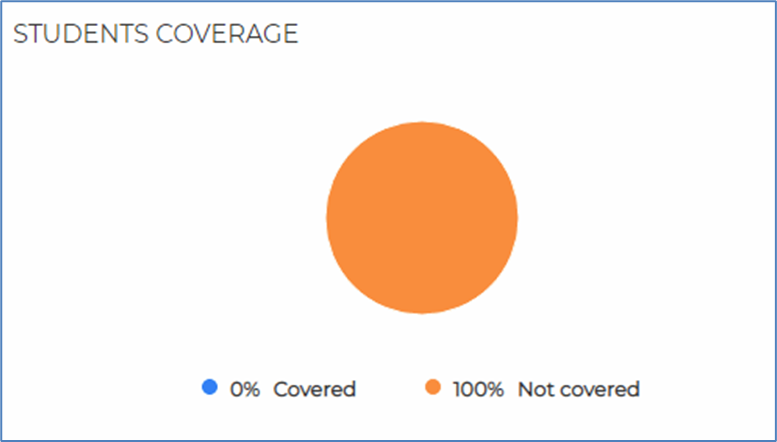This graph represents the percentage of the students, enrolled in the different courses, who checked the material mapped to learning objectives created on the same subject and grade as the courses where the material exist. The Covered percentage represents the percentage of students who checked the material mapped to learning objectives while the Not Covered percentage represents the percentage of students who did not check the material mapped to learning objectives.

Clicking the graph displays more information about the percentage of students who checked and did not check the material covered by learning objectives as in the following figure.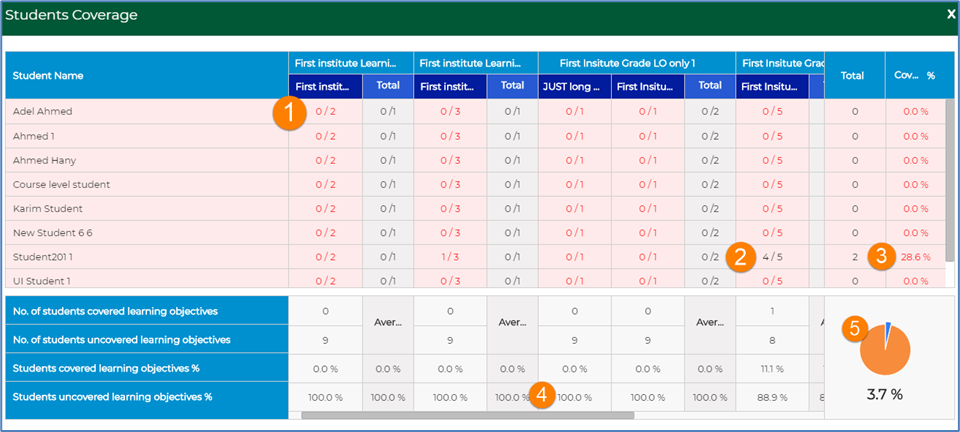The below table explains the numerical legend on the Students Coverage Details screen above.

 No. Explanation 1 For example, this student did not check any of the materials mapped to learning objectives. 2 For example, this student checked 4 out of the 5 material mapped to this learning objective. 3 This percentage represents the percentage of the total material checked by this student and that were mapped to learning objectives. 4 The average represents the number of students who checked material mapped to learning objectives divided by the total number of students enrolled in the courses. 5 Represents the total percentage of students who checked material mapped to learning objectives out of the total number of students enrolled in all the courses.

#### Students Achievements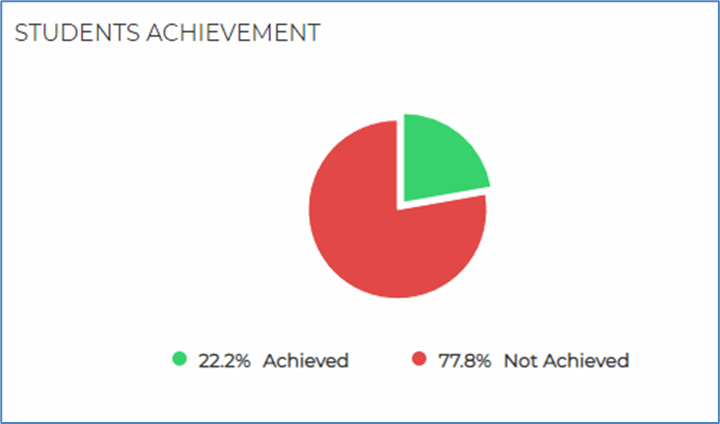This graph represents the percentage of the students, enrolled in the different courses, who solved questions mapped to learning objectives created on the same subject and grade as the courses where the questions exist. The Covered percentage represents the percentage of students who solved questions mapped to learning objectives while the Not Covered percentage represents the percentage of students who did not solve questions mapped to learning objectives.

Clicking the graph displays more information about the percentage of students who solved and did not solve questions mapped to learning objectives as in the following figure.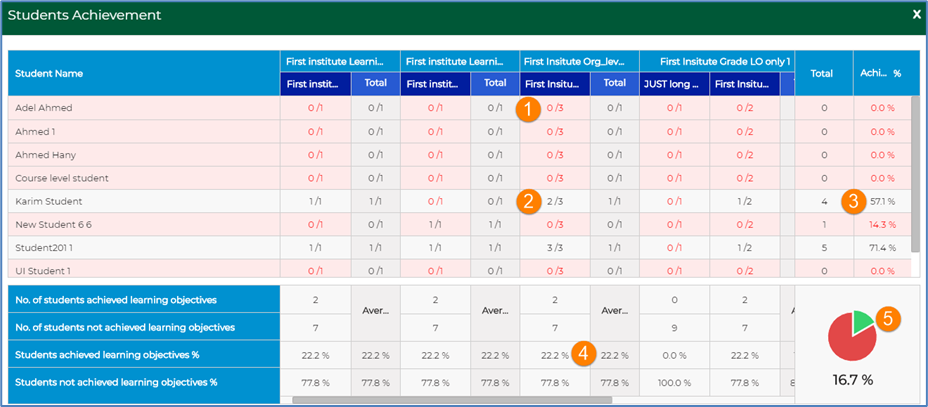The below table explains the numerical legend on the Students Achievements Details screen above.

 No. Explanation 1 For example, this student did not solve any of the 3 questions mapped to this learning objective. 2 For example, this student solved 2 out of the 3 questions mapped to this learning objective. 3 This percentage represents the percentage of the total questions solved by this student and that were mapped to learning objectives in proportion to the total number of questions mapped to learning objectives. 4 The average represents the number of students who solved questions mapped to learning objectives divided by the total number of students enrolled in the courses. 5 Represents the total percentage of students who solved questions mapped to learning objectives out of the total number of students enrolled in all the courses.